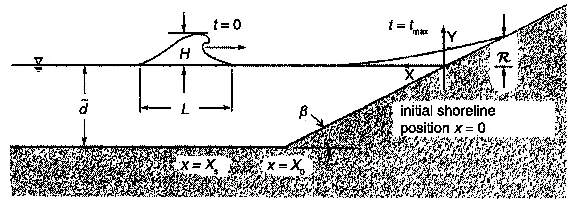Initial Value Problem

## Analytical benchmarking

The shallow water-wave (SW) equations describe the evolution of the water-surface elevation and of the depth-averaged water particle velocity of waves with wavelengths large compared with the depth of propagation. The equations assume that the pressure distribution is hydrostatic everywhere, i.e., there is no variation with depth of any of the other flow variables. In this section we present several analytic solutions to the 1+1 version of these equations. As stated in Section 2 of this report, 1+1 models are generally unreliable for inundation mapping and entirely inadequate for operational tsunami forecasting, but they are invaluable to the process of testing and validating models.